# Determination of Focal Length Power of Convex Lens by Auxiliary Lens Method.

\${tocify}

## Abstract

As a concave lens always forms a virtual image, its focal length cannot be found directly as for a convex lens. For this purpose, indirect method is used, as described below.

An object needle O is placed on one side of a convex lens L1 and its real inverted image I is located (by image needle) on the other side as shown in ray diagram.

The concave lens L2 is placed between convex lens L1 and image needle I. The concave lens diverges the rays and the image is now formed at l' as shown in ray diagram.

For concave lens, I is the virtual object and I' is the real image. Hence, O₂l = u and O₂l' = v.

Focal length can be calculated, using lens formula,

1/f = 1/v - 1/u

## Introduction

A lens is a transmissive optical device that focuses or disperses a light beam by means of refraction. A simple lens consists of a single piece of transparent material, while a compound lens consists of several simple lenses (elements), usually arranged along a common axis .

Lens are generally mainly two in number. They are-
1. Convex lens and
2. Concave lens.
A lens is biconvex (or double convex, or just convex) if both surfaces are convex. If both surfaces have the same radius of curvature, the lens is equiconvex. A lens with two concave surfaces is biconcave (or just concave). If one of the surfaces is flat, the lens is plano-convex or plano-concave depending on the curvature of the other surface. A lens with one convex and one concave side is convex-concave or meniscus .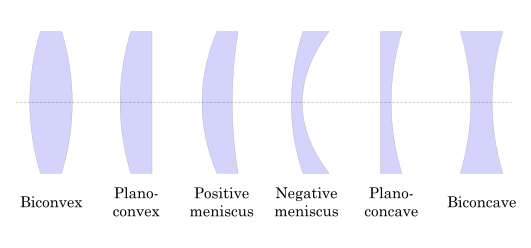Fig. 01 (types of lens)

### Convex lens

Convex lenses are thicker at the middle. Rays of light that pass through the lens are brought closer together (they converge).
A convex lens is a converging lens. When parallel rays of light pass through a convex lens the refracted rays converge at one point called the principal focus.
The distance between the principal focus and the centre of the lens is called the focal length .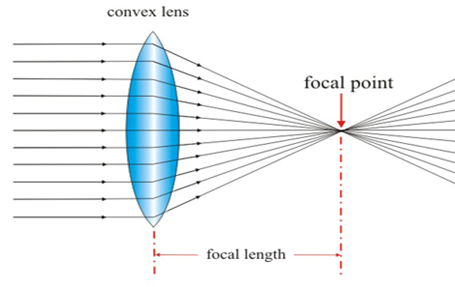Fig. 02 (convex lens)

### Concave lens

Concave lenses are thinner at the middle. Rays of light that pass through the lens are spread out (they diverge). A concave lens is a diverging lens.
When parallel rays of light pass through a concave lens the refracted rays diverge so that they appear to come from one point called the principal focus.
The distance between the principal focus and the centre of the lens is called the focal length.
The image formed is virtual and diminished (smaller) .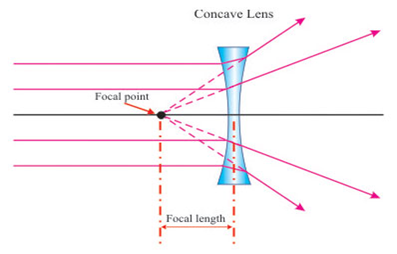Fig. 03 (concave lens)

### Auxiliary lens

A lens attached to an objective to change its focal length. A positive lens decreases the focal length, and a negative lens increases it. Positive lenses are used for largescale photography (macrophotography) with still and motion picture cameras, in which extension of the lens toward the object being photographed is limited.

The concave lens can not produce a real image of a real object; but if a virtual object is placed within its focus, it can produce a real image of the virtual object. This principle is utilized in determining the focal length of a concave lens. At first a real image of a real object is produced with the help of a convex lens. Then a convex lens is interposed between the convex lens and its real image in such a way that the real image falls within the focus of the concave lens.

## Theory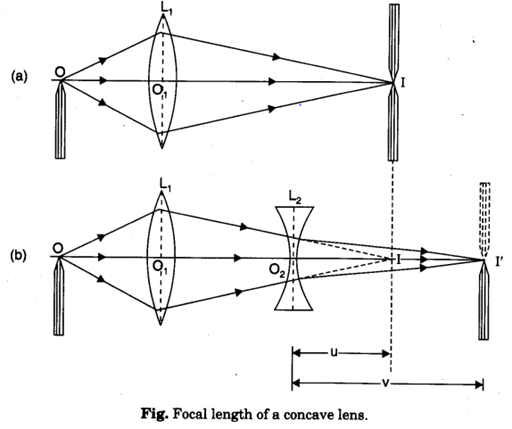From lens formula, We have,
f = (uv)/(u-v)… … … … … … … … … … … … (1)

Where,
f = focal length of concave lens L_2
U = distance of I from optical centre of lens L_2
V = distance of Iꞌ from optical centre of lens L_2
(Note. According to sign convention, u and v have positive values (being measured in direction of incident light. v < u, u – v is negative. Hence f comes negative)

And,
P = 100/f (cm) … … … … … … … … … … … … (2)

Where,  P = power
f =  focal length.

## Apparatus

1. Optical bench
2. Concave lens
3. Convex lens
4. Screen
5. Index rod etc.

## Experimental data

 No  Of object Position of Apparent Object distance U=L~P Apparent Image distance V=L~I U= U+λ V= V+ λ Object (o) Convex Lens (p) Image with   convex lens(p) Concave lens (L) Image with combination (I) 1 0 17 37 28.5 42 8.5 13.35 8.9 13.9 2 0 17 37 26 47 13 21 13.4 21.4 3 0 17 37 24.2 52 15 28 15.4 28.4 4 0 17 37 23.7 57 13 33.3 13.7 33.7

### Table: determining of ‘f’

 No Of object Object distance (v) Image distance (v) Focal length Mean focal length (f) cm Power,(p) =100/f(cm) diopter 1 8.9 13.9 -24.742 -26.82 -3.728 2 13.4 21.4 -25.845 3 15.4 28.4 -33.643 4 13.7 33.7 -23.084

### Table for index error ( λ ) between convex lens & screen

 Length index rod (cm) l Diff. of bench scale reading in cm when the two ends of the index rod touch the concave lens & screen Index correction in cm  λ =(l-d) 30.3 29.9 0.4

## Calculation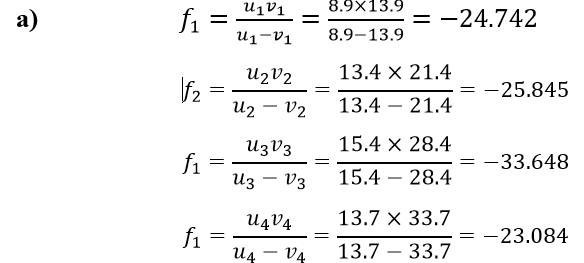Focal length ,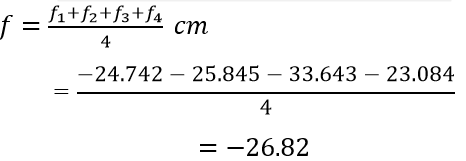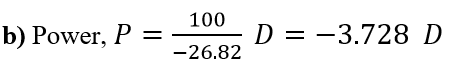## Percentage of Error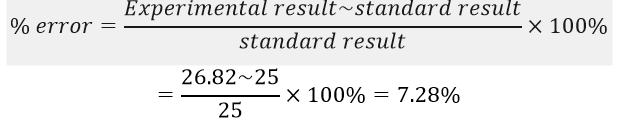## Result

The focal length of the given concave lens is -26.82 cm. with an error of 7.28% and power is -3.728 D

## Discussion

The image formed by the concave lens was focused on the screen by shifting the positions of the concave lens and not by moving the screen. This is necessary because the focused condition of the image would not change within an appreciable range of the movement of the screen.
The light emerges from the concave lens parallel to the axis and consequently no image is formed when LP is equal to the focal length of the concave lens.
Knowing the distances of an object and distance of image formed, we can calculate every accurate focal length. Our focal length were not very close enough and especially the first is too small. However those readings were taken more carefully and accurately.

## Conclusion

In this experiment we had determined the focal length of given concave lens and its power. During experiment, it was necessary to take position of lens by a distance of 4f for concave lens.

## References

. http://www.passmyexams.co.uk/GCSE/physics/concave-lenses-convex-lenses.html
. http://www.passmyexams.co.uk/GCSE/physics/concave-lenses-convex-lenses.html

Tags: focal length and power of convex lens, focal length and power of convex lens experiment, find the focal length and power of a convex lens, focal point of a convex lens, power of convex lens with focal length, lens equation focal length,calculate focal length plano convex lens, focal length plano convex lens